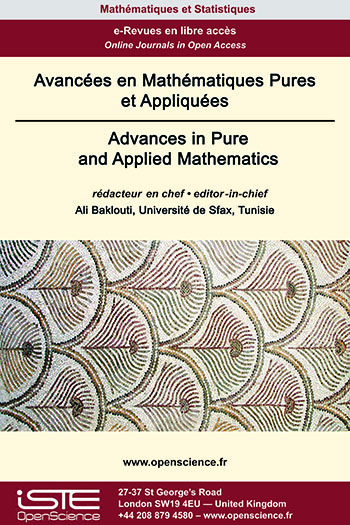# Vol 14 - Issue 2 (Special CSMT 2022)

## List of Articles

Extension of Semistar Operations

Let RT be an extension of integral domains and ∗ be a semistar operation stable of finite type on R. We define a semistar operation ∗1 on T in the following way: for each nonzero T-submodule E of the quotient field K1 of T, let E∗1 = ∪ {E :K1 JT | J ∈ $\mathcal{F}$∗}, where K1 denotes the quotient field of T and $\mathcal{F}$∗ the localizing system associated to ∗. In this paper we investigate the basic properties of ∗1. Moreover, we show that the map $\varphi$ which associates to a semistar operation ∗ stable and of finite type on R, the semistar operation ∗1 is continuous. Furthermore, we give sufficient conditions for $\varphi$ to be a homeomorphism.

Bifurcation beyond the principal eigenvalues for Neumann problems with indefinite weights

This paper is devoted to the study of the effects of indefinite weights on the following nonlinear Neumann problems
${(P^\pm)} \begin{cases} -\Delta u &= \lambda \, a(x) u \pm |u|^{p-1}u\ &\quad \hbox{in } \Omega \subset ℝ^N \\ \frac{\partial u}{\partial \nu} &=\ 0 \ & \hbox{on } \partial \Omega \end{cases}$
The function $a = a(x)$ is assumed to be continuous and sign-changing. Then the linear part has two sequences of eigenvalues. Our results establish a relation between the position of the parameter $\lambda$ and the number of nontrivial classical solutions of these problems. The proof combines spectral analysis tools, variational methods and the Clark multiplicity theorem.

Topological invariants for the scalar curvature problem on manifolds

In , A.Bahri introduced two topological invariants μ and τ to study the prescribed scalar curvature problem on standard spheres of high dimensions. In this paper we first extend μ and τ to the problem on general riemannian manifolds. Second we analyze, as suggested in , the relation between these two quantities and we prove under topological conditions that μ = τ.

Gysin-(ℤ/2ℤ)d-functors

Let d ≥ 1 be an integer and Kd be a contravariant functor from the category of subgroups of (ℤ/2ℤ)d to the category of graded and finite 𝔽2-algebras. In this paper, we generalize the conjecture of G. Carlsson [C3], concerning free actions of (ℤ/2ℤ)d on finite CW-complexes, by suggesting, that if Kd is a Gysin-(ℤ/2ℤ)d-functor (that is to say, the functor Kd satisfies some properties, see 2.2), then we have:
$\big(C_{d} \big): \; \underset{i \geq 0}{\sum}dim_{\mathbb{F}_{2}} \big(\mathcal{K}_{d}(0)\big)^{i} \geq 2^{d}$
We prove this conjecture for 1 ≤ d ≤ 3 and we show that, in certain cases, we get an independent proof of the following
results (for d = 3 see [C4]):
If the group (ℤ/2ℤ)d, 1 ≤ d ≤ 3, acts freely and cellularly on a finite CW-complex X, then ${\underset{i \geq 0}{\sum}}dim_{\mathbb{F}_{2}}H^{i}(X;\; \mathbb{F}_{2}) \geq 2^{d}$### Other issues :

2020

Volume 20- 11

Issue 1 (May 2020)
Issue 2 (September 2020)

2021

Volume 21- 12

Issue 1 (January 2021)
Issue 2 (May 2021)
Issue 3 (Special AUS-ICMS 2020)
Issue 4 (September 2021)

2022

Volume 22- 13

Forthcoming papers

Issue 1 (January 2022)
Issue 2 (March 2022)
Issue 3 (June 2022)
Issue 4 (September 2022)

2023

Volume 23- 14

Issue 1 (January 2023)
Issue 2 (Special CSMT 2022)